# Function

This is the current revision of Function as edited by at 13:13, July 13, 2016. This URL is a permanent link to this version of this page.

(diff) ← Older revision | Latest revision (diff) | Newer revision → (diff)
Jump to: navigation, search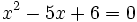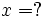This article/section deals with mathematical concepts appropriate for a student in mid to late high school.

A function "f" is a fixed method for calculating a unique output "f(x)" for every given input "x". For example, the polynomial function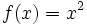takes numbers as inputs and outputs their squares. So in this case, if 1 is input, 1 is output, whilst if 2 is input, 4 is output.

A function is typically written with a formula for the output, in terms of the input. For example, in the case of f above, the formula is. When we want to input a particular value, we insert it in the place of x. So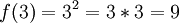.

## Examples

If the inputs and outputs are numbers, then the most obvious examples are the polynomials. f(x) = x + 1 is a simple example, a function that adds one to the input. f(1) = 1 + 1 = 2, f(2) = 2 + 1 = 3, and so on. Also, g(x) = 2x is a function that doubles the input. g(3) = 6, g(4) = 8. Another example is h(x) = 2x + 1. This doubles the output, and adds 1. The result is that h(1) = 2*1 + 1 = 3, h(2) = 2*2 + 1 = 5.

i(x) = x is another function that does nothing to x. So i(1) = 1, i(2) = 2, and so on. This is called the identity function.

Some functions do not have numbers as outputs. For example, we can define a function "pres" that takes a number n, and returns the name of the nth American President. So, pres(1) = George Washington (the first president), pres(2) = John Adams (the second president), and so on.

Similarly, not all functions take numbers as inputs. For example, we could define a function "presnumber" that takes the name of an American President (the nth in sequence), and outputs n. Thus presnumber(George Washington) = 1, presnumber(John Adams) = 2. This would be the inverse of pres defined above. (However, due to a historical curiosity, this function is not properly defined; see below.)

## Some Properties and Terminology

The set of possible inputs is called the domain of the function. It is usually a set of numbers, such as the set of all real numbers. The set of all possible outputs is called the range. It, too, is often the set of real numbers. A function might not give result values for all possible items in the range. The set of items in the range that are possible values is called the image. For example, the functionhas the real numbers as its domain and range, but its image is just the non-negative numbers.

A function must be "single-valued", that is, have a rule that uniquely gives its result for any input. The "presnumber" function that we attempted to define above fails, because presnumber(Grover Cleveland) is not properly defined.

If a function can give as output every item in the range, it is said to be onto or surjective. That is, a function is onto if its image is the same as its range.

If a function has only one unique input that yields any given output, it is said to be one-to-one or injective.

A function that is both surjective and injective is daid to be a one-to-one correspondence, or bijective. For such a function, there is a unique correspondence between every point in the domain and every point in the range.

The functionfails to be onto because there is no input that gives a result of, say, -3. It fails to be one-to-one becauseand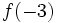are the same number.

The fact that a function is a one-to-one correspondence does not mean that its domain and image are the same. There is, for example, a one-to-one correspondence between the set of all reals and the interval. The arctangent function does it.

## Composition

We can take 2 functions, and combine them to form another function, by applying them one after another. This is called composition. The notation for this is a small circle written between the two function names. Beware!! The function to the right of the circle is evaluated first, followed by the function to the left. For example, take f(x) = x + 1, and g(x) = 2x. We can apply g to x, and then f to the output g(x), to get f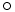g, which is the function we get by combining the functions f and g in this way. We can think of this as like a factory production line, where the output of one process or machine becomes the input of the next. Here, the output of g becomes the input of the function f.

In our example, (fg)(x) = f(g(x)) = f(2x) = 2x + 1. So the combined function fg is in fact h, which we met earlier. To test this, we can take (fg) applied to 8. g(8) = 2*8 = 16. Then we apply f to 16, to get f(16) = 16 + 1 = 17. 17 = 2*8 + 1, as expected.

We can also apply the functions in the opposite order to get gf, where we apply f first then g. f(x) = x + 1, and g(x+1) = 2(x+1) = 2x + 2. So (gf)(x) = 2x + 2. So, the order in which we combine the functions makes a difference to the final result. Again, we test this formula by applying the function to 8. f(8) = 9, and g(9) 18. 18 = 2*8 + 2, as expected.

## Non-numeric functions

There are functions that take objects other than numbers as inputs. For example, rotation can be thought of as a function that takes a shape as an input, and outputs that shape rotated by a certain angle.